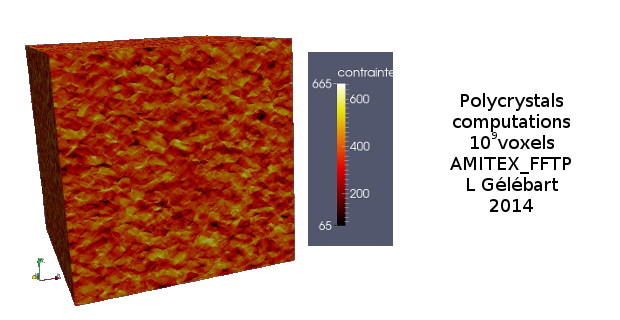•The amitex interface is mostly an alias for the castem interface, i.e. the interface for the Cast3M interface prior to Version 21 and also generates an input file template which is meant to be copy-pasted into the material.xml file of the user. See this page for a description of the castem interface

# Generated input file template

Here is an example of such a template:

<!--
Simple template for use in AMITEX_FFTP.

You may copy-paste those lines in your material.xml file

- the material number I,
- the path to the library (currently UmatBehaviour),
- the coefficients and initial internal variables values
which are currently replaced by three dots.
-->

<Material numM="I" Lib="UmatBehaviour" Law="umatplasticity">
<!-- material property YoungModulus -->
<Coeff Index = "1" Type = "Constant" Value = "..." />
<!-- material property PoissonRatio -->
<Coeff Index = "2" Type = "Constant" Value = "..." />
<!-- material property MassDensity -->
<Coeff Index = "3" Type = "Constant" Value = "..." />
<!-- material property ThermalExpansion -->
<Coeff Index = "4" Type = "Constant" Value = "..." />
<!-- material property H -->
<Coeff Index = "5" Type = "Constant" Value = "..." />
<!-- material property s0 -->
<Coeff Index = "6" Type = "Constant" Value = "..." />
<!-- internal state variable ElasticStrain -->
<IntVar Index = "1" Type = "Constant" Value = "..." />
<IntVar Index = "2" Type = "Constant" Value = "..." />
<IntVar Index = "3" Type = "Constant" Value = "..." />
<IntVar Index = "4" Type = "Constant" Value = "..." />
<IntVar Index = "5" Type = "Constant" Value = "..." />
<IntVar Index = "6" Type = "Constant" Value = "..." />
<!-- internal state variable EquivalentPlasticStrain -->
<IntVar Index = "7" Type = "Constant" Value = "..." />
</Material>